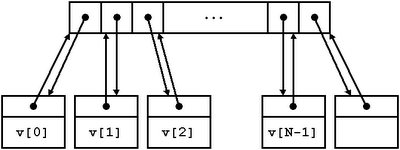## Friday, August 29, 2008

### Stable vectors

Extremely convenient as they are, `std::vector`s have a limitation that many novice C++ programmers frequently stumble upon: iterators and references to an element of an `std:vector` are invalidated when a preceding element is erased or when the vector expands and needs to migrate its internal storage to a wider memory region (i.e. when the required size exceeds the vector's capacity). We say then that `std::vector`s are unstable: by contrast, stable containers are those for which references and iterators to a given element remain valid as long as the element is not erased: examples of stable containers within the C++ standard library are `std::list` and all the associative containers.

Sometimes stability is too precious a feature to live without. One particular property of `std::vector`s, namely element contiguity, makes it impossible to add stability to this container. So, provided we sacrifice element contiguity, how much can a stable design approach the behavior of `std::vector` (random access iterators, amortized constant time end insertion/deletion, minimal memory overhead, etc.)?

The following image describes the layout of a possible data structure upon which to base the design of a stable vector:Each element is stored in its own separate node. All the nodes are referenced from a contiguous array of pointers, but also every node contains an "up" pointer referring back to the associated array cell. This up pointer is the key element to implementing stability and random accessibility:

1. Iterators point to the nodes rather than to the pointer array. This ensures stability, as it is only the pointer array that needs to be shifted or relocated upon insertion or deletion.
2. Random access operations can be implemented by using the pointer array as a convenient intermediate zone. For instance, if the iterator `it` holds a node pointer `it.p` and we want to advance `it` by `n` positions, we simply do:
`‎it.p = *(it.p->up+n);`
That is, we go "up" to the pointer array, add `n` there and then go "down" to the resulting node.

In a later entry we will present a full implementation of an STL-conformant stable vector and compare its characteristics (space/time complexity, exception safety) with those of `std::vector`.

## Monday, August 25, 2008

### Cpp bignum arithmetic with memoization

The multiplication algorithm we implemented for C preprocessor bignums incurs a certain amount of repetitive work: multiplying `bn1` by `bn2` = `bn21 bn22···bn2m`, involves calculating the set of intermediate products `bn1`·`bn21`, ... ,`bn1`·`bn2m`, which includes as many repeated values as equal digits are in the decimal expansion of `bn2`. We can avoid recalculating those by applying memoization to the `BN_DIGIT_MUL` macro:

`‎#define BN_CACHED_DIGIT_MUL(bn,y,cache) \‎BOOST_PP_IF( \‎  BOOST_PP_EQUAL( \‎    1, \‎    BOOST_PP_SEQ_SIZE(BOOST_PP_SEQ_ELEM(y,cache))), \‎  BN_CACHED_DIGIT_MUL_CASE1, \‎  BN_CACHED_DIGIT_MUL_CASE2)(bn,y,cache)‎‎#define BN_CACHED_DIGIT_MUL_CASE1(bn,y,cache) \‎BN_CACHED_DIGIT_MUL_CASE1_IMPL(BN_DIGIT_MUL(bn,y),y,cache)‎‎#define BN_CACHED_DIGIT_MUL_CASE1_IMPL(bny,y,cache) \‎(bny)(BOOST_PP_SEQ_REPLACE(cache,y,(~)bny))‎‎#define BN_CACHED_DIGIT_MUL_CASE2(bn,y,cache) \‎(BOOST_PP_SEQ_TAIL(BOOST_PP_SEQ_ELEM(y,cache)))(cache)`

`BN_CACHED_DIGIT_MUL(bn,y,cahe)` accepts a cache memory in the form of a Boost.Preprocessor sequence storing those of the values `bn`·0, `bn`·1, ..., `bn`·9 calculated so far (with a special format not worth discussing in detail here). If the cache already has the requested multiplication, then this is used, otherwise we calculate the value using `BN_DIGIT_MUL` and update the cache accordingly. The return value is a pair containing both the result and the updated cache, which can be passed later to subsequent invocations to `BN_CACHED_DIGIT_MUL`. To make `BN_MUL` benefit from the usage of `BN_CACHED_DIGIT_MUL` we have to add the corresponding cache as an additional member of the state structure that constitutes the core of the multiplication algorithm.

`‎‎#define BN_MUL(bn1,bn2) \‎BN_MUL_STATE_RESULT( \‎  BOOST_PP_SEQ_FOLD_LEFT( \‎    BN_MUL_OP, \‎    ((~))(bn2) \‎    (((~)(0))((~)bn2)((~))((~))((~))((~))((~))((~))((~))((~))) \‎    ((0)), \‎    BOOST_PP_SEQ_REVERSE(bn1)))‎‎#define BN_MUL_OP(s,state,x) \‎BN_MUL_OP_IMPL( \‎  x, \‎  BN_MUL_STATE_SHIFT(state), \‎  BN_MUL_STATE_BN2(state), \‎  BN_MUL_STATE_CACHE(state), \‎  BN_MUL_STATE_RESULT(state))‎‎#define BN_MUL_OP_IMPL(x,shift,bn2,cache,result) \‎BN_MUL_OP_IMPL_RETURN( \‎  BN_CACHED_DIGIT_MUL(bn2,x,cache),shift,bn2,result)‎‎#define BN_MUL_OP_IMPL_RETURN(bn2x_cache,shift,bn2,result) \‎(shift(0)) \‎(bn2) \‎(BOOST_PP_SEQ_ELEM(1,bn2x_cache)) \‎(BN_ADD( \‎   result, \‎   BOOST_PP_SEQ_ELEM(0,bn2x_cache)BOOST_PP_SEQ_TAIL(shift)))‎‎#define BN_MUL_STATE_SHIFT(state) BOOST_PP_SEQ_ELEM(0,state)‎#define BN_MUL_STATE_BN2(state) BOOST_PP_SEQ_ELEM(1,state)‎#define BN_MUL_STATE_CACHE(state) BOOST_PP_SEQ_ELEM(2,state)‎#define BN_MUL_STATE_RESULT(state) BOOST_PP_SEQ_ELEM(3,state)`

The initial cache value is marked in red above, note that it already contains precomputed values for the easy cases `bn2`·0 and `bn2`·1.

We can also apply memoization to the implementation of `BN_DIV`: the macro `BN_QDIGIT_REMAINDER(bn1,bn2)`, which returns the largest decimal digit `q` such that `bn2`·`q``bn1`, along with `bn1``bn2`·`q`, can be supplemented with a cache to store the values `bn`2·0, `bn2`·1, ..., `bn`2·9. The implementation details are entirely similar to those involved in memoizing `BN_MUL`. A full implementation of the memoized bignum arithmetic operations is provided.

Let us measure the improvement brought about by memoization. These 10-, 20- and 30-digit random numbers were selected with the aid of random.org:

n1 = 8579726378,
n2 = 88242457695260718048,
n3 = 7278842148,
n4 = 17478148223952531376,
n5 = 22737602045089519821,
n6 = 859621743146723715335628844223,
n7 = 97249055978154178611,
n8 = 502003375419877211491785910042,

and the following operations were timed for the plain and memoized versions of bignum multiplication and division macros; figures are averages of several individual measurements, tests were run with GCC 3.4.4 for Cygwin in a Windows box with a 1.66 GHz Intel Core 2 Duo and 1 GB RAM:

 operation plain version(s) memoized version(s) speedupfactor n1 · n2 11.2 9.1 19% n2 · n1 13.3 9.7 27% n3 · n4 9.8 7.0 28% n4 · n3 12.0 9.7 19% n5 · n6 27.2 20.6 24% n6 · n5 30.0 22.3 25% n7 · n8 27.9 19.9 28% n8 · n7 31.7 22.0 30% n2 / n1 8.9 7.8 13% n4 / n3 5.9 4.9 17% n6 / n5 11.7 9.5 19% n8 / n7 11.2 9.8 12%

The speedup factor is defined as (pm)/p, where p is the time taken by the plain version and m the time with memoization. Improvements are noticeable though not dramatic. Multiplication could be further accelerated by using alternative algorithms like for instance Karatsuba's, but we decide to leave it at this for the moment.